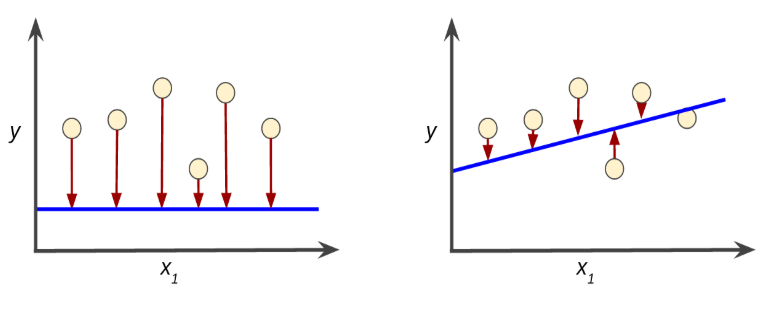+1 vote
6 views

While training my neural network using Theano or tensorflow, a variable called “loss” per epoch was reported. Now the question is, How to interpret this variable? What does it mean for the accuracy of my neural network?

by (10.9k points)

A loss function is used to optimize a machine learning algorithm. The loss is calculated on training and validation and its interpretation is based on how well the model is doing in these two sets. It is the sum of errors made for each example in training or validation sets. Loss value implies how poorly or well a model behaves after each iteration of optimization.

An accuracy metric is used to measure the algorithm’s performance in an interpretable way. The accuracy of a model is usually determined after the model parameters and is calculated in the form of a percentage. It is the measure of how accurate your model's prediction is compared to the true data.

Example-
Suppose you have 1000 test samples and if your model is able to classify 990 of them correctly, then the model’s accuracy will be 99.0%.

If You want to learn Machine Learning, visit this machine learning interview questions and machine learning tutorial for better understanding.

You should also, watch this Machine Learning tutorial to understand the concept:

by (108k points)
edited by

Training a model simply means learning (determining) good values for all the weights and the bias from labeled examples.

Loss is the result of a bad prediction. A loss is a number indicating how bad the model's prediction was on a single example.

If the model's prediction is perfect, the loss is zero; otherwise, the loss is greater. The goal of training a model is to find a set of weights and biases that have low loss, on average, across all examples. Higher loss is the worse(bad prediction) for any model.

The loss is calculated on training and validation and its interpretation is how well the model is doing for these two sets. Unlike accuracy, a loss is not a percentage. It is a sum of the errors made for each example in training or validation sets.

In the following diagrams, there are two graphs representing the losses of two different models, the left graph has a high loss and the right graph has a low loss.• The arrows represent a loss.

• The blue lines represent predictions.

Hope this helps!

If you want to know more about Machine Learning then watch this video: